# ‘MCMCvis’ package

## Intro

`MCMCvis` is an R package used to visualize, manipulate, and summarize MCMC output. This may be Bayesian model output fit with Stan, NIMBLE, JAGS, and other software.

The package contains six functions:

• `MCMCsummary` - summarize MCMC output for particular parameters of interest
• `MCMCpstr` - summarize MCMC output and extract posterior chains for particular parameters of interest while preserving parameter structure
• `MCMCtrace` - create trace and density plots of MCMC chains for particular parameters of interest
• `MCMCchains` - extract posterior chains from MCMC output for particular parameters of interest
• `MCMCplot` - create caterpillar plots from MCMC output for particular parameters of interest
• `MCMCdiag` - create a `.txt` file and save specified objects that summarize model inputs, outputs, and diagnostics

`MCMCvis` was designed to perform key functions for MCMC analysis using minimal code, in order to free up time/brainpower for interpretation of analysis results. Functions support simple and straightforward subsetting of model parameters within the calls, and produce presentable, ‘publication-ready’ output.

All functions in the package accept `stanfit` objects (created with the `rstan` package), `CmdStanMCMC` objects (created with the `cmdstanr` package), `stanreg` objects (created with the `rstanarm` package), `brmsfit` objects (created with the `brms` package), `mcmc.list` objects (created with the `rjags` or `coda` packages), `mcmc` objects (created with the `coda` or `nimble` packages), `list` objects (created with the `nimble` package), `R2jags` output (created with the `R2jags` package), `jagsUI` output (created with the `jagsUI` package), and matrices of MCMC output (chains combined into a single column per parameter - columns to be named with parameter names). The functions automatically detect the object type and proceed accordingly. Model output objects can be inserted directly into the `MCMCvis` functions as an argument.

#### JAGS model

``````library(rjags)

# create JAGS model
mf <- "
model {
for (i in 1:10)
{
y[i] ~ dnorm(mu, 0.01);
}
mu ~ dnorm(0, 0.01)
}
"

data <- list(y = rnorm(10))

jm <- rjags::jags.model(
textConnection(mf),
data = data,
n.chains = 3)

jags_out <- rjags::coda.samples(
jm,
variable.names = 'mu',
n.iter = 500)``````
``library(MCMCvis)``
``MCMCsummary(jags_out, round = 2)``
``````##     mean   sd  2.5%   50% 97.5% Rhat n.eff
## mu -0.28 2.97 -6.13 -0.14  5.22    1  1397``````

#### NIMBLE model

``````library(nimble)

# create NIMBLE model
mf2 <- nimbleCode({
for (i in 1:10) {
y[i] ~ dnorm(mu, 0.01);
}
mu ~ dnorm(0, 0.01)
})

nimble_out <- nimbleMCMC(
code = mf2,
constants = list(N = 10),
data = data,
inits = list(mu = 0),
nchains = 4,
niter = 500)``````
``MCMCsummary(nimble_out, round = 2)``
``````##     mean   sd  2.5%   50% 97.5% Rhat n.eff
## mu -0.03 2.99 -5.76 -0.1  5.88    1  2000``````

#### Stan model

``````library(rstan)

# create Stan model
sm <- "
data {
real y;
}
parameters {
real mu;
}
model {
for (i in 1:10) {
y[i] ~ normal(mu, 10);
}
mu ~ normal(0, 10);
}
"

stan_out <- stan(
model_code = sm,
data = data,
iter = 500)``````
``MCMCsummary(stan_out, round = 2)``
``````##       mean   sd  2.5%   50% 97.5% Rhat n.eff
## mu   -0.51 2.82 -6.06 -0.36  5.07 1.01   414
## lp__ -0.45 0.61 -2.27 -0.23 -0.01 1.00   508``````

## MCMCsummary

`MCMCsummary` is used to output summary information from MCMC output as a data.frame. All digits are reported by default.

We’ll use the built in `mcmc.list` object for the examples below, but model output of any of the supported types will behave in the same way.

``MCMCsummary(MCMC_data)``
``````##                mean        sd       2.5%        50%      97.5% Rhat n.eff
## alpha  -9.775030  2.087347 -13.873736  -9.747235  -5.718132    1 10406
## alpha -10.954077  4.087058 -18.986880 -10.996852  -2.932785    1 10807
## alpha -12.812863  5.345266 -23.334561 -12.833156  -2.505842    1 10500
## alpha -13.162363  4.549087 -22.002841 -13.131785  -4.270300    1 11160
## alpha -11.683834  8.888936 -28.857696 -11.734387   6.059936    1 10253
## alpha  -8.272228  6.200246 -20.263314  -8.218744   3.889713    1 10500
## beta   -4.618601  6.544247 -17.193329  -4.619954   8.378438    1 10411
## beta  -14.169569  6.633089 -27.152037 -14.084978  -1.414025    1 10500
## beta  -35.936571  8.415110 -52.599736 -35.998599 -19.259210    1 10884
## beta    6.172961 10.716345 -14.670444   6.110057  27.266086    1 10500
## beta    8.423471  3.463758   1.625696   8.447591  15.125755    1 10500
## beta  -12.051330  2.336788 -16.655170 -12.046641  -7.537826    1 10500``````

The number of decimal places displayed can be specified with `round` (except for Rhat which is always rounded to 2 decimal places and n.eff which always displays whole numbers). Alternatively, the significant digits displayed can be specified with `digits`.

``MCMCsummary(MCMC_data, round = 2)``
``````##            mean    sd   2.5%    50%  97.5% Rhat n.eff
## alpha  -9.78  2.09 -13.87  -9.75  -5.72    1 10406
## alpha -10.95  4.09 -18.99 -11.00  -2.93    1 10807
## alpha -12.81  5.35 -23.33 -12.83  -2.51    1 10500
## alpha -13.16  4.55 -22.00 -13.13  -4.27    1 11160
## alpha -11.68  8.89 -28.86 -11.73   6.06    1 10253
## alpha  -8.27  6.20 -20.26  -8.22   3.89    1 10500
## beta   -4.62  6.54 -17.19  -4.62   8.38    1 10411
## beta  -14.17  6.63 -27.15 -14.08  -1.41    1 10500
## beta  -35.94  8.42 -52.60 -36.00 -19.26    1 10884
## beta    6.17 10.72 -14.67   6.11  27.27    1 10500
## beta    8.42  3.46   1.63   8.45  15.13    1 10500
## beta  -12.05  2.34 -16.66 -12.05  -7.54    1 10500``````

Specific parameters can be specified to subset summary information. Square brackets in parameter names are ignored by default. For instance, all `alpha` parameters can be plotted using `params = 'alpha'`.

``````MCMCsummary(MCMC_data,
params = 'alpha',
round = 2)``````
``````##            mean   sd   2.5%    50% 97.5% Rhat n.eff
## alpha  -9.78 2.09 -13.87  -9.75 -5.72    1 10406
## alpha -10.95 4.09 -18.99 -11.00 -2.93    1 10807
## alpha -12.81 5.35 -23.33 -12.83 -2.51    1 10500
## alpha -13.16 4.55 -22.00 -13.13 -4.27    1 11160
## alpha -11.68 8.89 -28.86 -11.73  6.06    1 10253
## alpha  -8.27 6.20 -20.26  -8.22  3.89    1 10500``````

Individual parameters can also be specified. For example, one `alpha` (of many) may be specified. In this case, the square brackets should not be ignored, so that only the `alpha` parameter can be specified. Use the argument `ISB = FALSE` to prevent square brackets from being ignored (ISB is short for ‘Ignore Square Brackets’). The `exact` argument can be used to specify whether the parameter name should be matched exactly (after taking into account the `ISB` argument). Therefore, to return a single `alpha` parameter, `ISB = FALSE` and `exact = TRUE` can be used in the following way.

``````MCMCsummary(MCMC_data,
params = 'alpha',
ISB = FALSE,
exact = TRUE,
round = 2)``````
``````##           mean   sd   2.5%   50% 97.5% Rhat n.eff
## alpha -9.78 2.09 -13.87 -9.75 -5.72    1 10406``````

When `exact = FALSE`, the `params` argument reads like a regular expression. Because of this, square brackets must be escaped with `\\`. All other regular expression syntax is accepted, as typically applied in R. This can be useful for returning a specific set of a large number of parameters. A great tools for regular expressions in R can be found here (https://regexr.com/) (though note two slashes are needed to escape characters in this function rather than one, hence `\\[` as opposed to `\[`). `\\d` can be used to specify any digits in a particular place.

``````MCMCsummary(MCMC_data,
params = 'alpha\\[1\\]',
ISB = FALSE,
exact = FALSE,
round = 2)``````
``````##           mean   sd   2.5%   50% 97.5% Rhat n.eff
## alpha -9.78 2.09 -13.87 -9.75 -5.72    1 10406``````

When using `exact = FALSE`, if `alpha` has 10 or more parameters (i.e., more than two digits for the index), the `|` (OR) may be needed. For instance, while one could use `alpha[1:10]` to select the first ten indices of the vector `alpha` in R, the regex equivalent to do the same with `MCMCvis` would be `alpha\\[(\\d|)\\]`. The `\\d` specifies any digit, the `|` represents OR (so any one digit number OR), the `` specifies that the first digit must be one, and the `` specifies that the second digit must be zero (so return any one digit number, or 10, resulting in the equivalent of `alpha[1:10]`). Ranges for each digit can also be specified. For instance, the regex equivalent of the R `alpha[5:15]` would be `alpha\\[([5-9]|[0-5])\\]`.

The `excl` argument can be used to exclude any parameters. For instance, if all parameters are desired except for the `alphas`, use `params = 'all', excl = 'alpha', ISB = FALSE, exact = FALSE`. These arguments can be used in any of the functions in the package.

``````MCMCsummary(MCMC_data,
params = 'all',
excl = 'alpha',
ISB = FALSE,
exact = FALSE,
round = 2)``````
``````##           mean    sd   2.5%    50%  97.5% Rhat n.eff
## beta  -4.62  6.54 -17.19  -4.62   8.38    1 10411
## beta -14.17  6.63 -27.15 -14.08  -1.41    1 10500
## beta -35.94  8.42 -52.60 -36.00 -19.26    1 10884
## beta   6.17 10.72 -14.67   6.11  27.27    1 10500
## beta   8.42  3.46   1.63   8.45  15.13    1 10500
## beta -12.05  2.34 -16.66 -12.05  -7.54    1 10500``````

Setting the `Rhat` and `n.eff` arguments to `FALSE` can be used to avoid calculating the Rhat statistic and number of effective samples, respectively (defaults for both `Rhat` and `n.eff` are `TRUE`). Specifying `FALSE` may greatly increase function speed with very large `mcmc.list` objects. Values for Rhat near 1 suggest convergence (Brooks and Gelman 1998). Rhat and n.eff values for `mcmc.list` objects are calculated using the `coda` package (what is typically returned by packages that utilize JAGS). Rhat and n.eff values for `stanfit` and `jagsUI` objects are calculated using a ‘split chain’ Rhat (as used by their respective packages). The approaches differ slightly between the `coda` and `stanfit`/`jagsUI` packages. Details on calculation of Rhat and number of effective samples using `rstan` can be found in the Stan manual (Stan Development Team 2018).

``````MCMCsummary(MCMC_data,
params = 'alpha',
Rhat = TRUE,
n.eff = TRUE,
round = 2)``````
``````##            mean   sd   2.5%    50% 97.5% Rhat n.eff
## alpha  -9.78 2.09 -13.87  -9.75 -5.72    1 10406
## alpha -10.95 4.09 -18.99 -11.00 -2.93    1 10807
## alpha -12.81 5.35 -23.33 -12.83 -2.51    1 10500
## alpha -13.16 4.55 -22.00 -13.13 -4.27    1 11160
## alpha -11.68 8.89 -28.86 -11.73  6.06    1 10253
## alpha  -8.27 6.20 -20.26  -8.22  3.89    1 10500``````

Sample quantiles in MCMCsummary can now be specified directly using the `probs` argument, removing the need to define custom quantiles with the `func` argument. The default behavior is to provide 2.5%, 50%, and 97.5% quantiles. These probabilities can be changed by supplying a numeric vector to the `probs` argument.

``````MCMCsummary(MCMC_data,
params = 'alpha',
Rhat = TRUE,
n.eff = TRUE,
probs = c(0.1, 0.5, 0.9),
round = 2)``````
``````##            mean   sd    10%    50%   90% Rhat n.eff
## alpha  -9.78 2.09 -12.47  -9.75 -7.13    1 10406
## alpha -10.95 4.09 -16.18 -11.00 -5.67    1 10807
## alpha -12.81 5.35 -19.76 -12.83 -6.10    1 10500
## alpha -13.16 4.55 -19.06 -13.13 -7.29    1 11160
## alpha -11.68 8.89 -23.03 -11.73 -0.36    1 10253
## alpha  -8.27 6.20 -16.34  -8.22 -0.31    1 10500``````

Setting `HPD = TRUE` will cause MCMCsummary to use `HPDinterval` from the `coda` package to compute highest posterior density intervals based on the probability specified in the `hpd_prob` argument (this argument is different than `probs` argument, which is reserved for quantiles). Note that for each parameter `HPDinterval` normally returns one interval per chain. However, MCMCsummary first pools the chains, forcing `HPDinterval` to compute a single interval across all posterior samples for each parameter. This step is done for user convenience.

``````MCMCsummary(MCMC_data,
params = 'alpha',
Rhat = TRUE,
n.eff = TRUE,
HPD = TRUE,
hpd_prob = 0.8,
round = 2)``````
``````##            mean   sd 80%_HPDL 80%_HPDU Rhat n.eff
## alpha  -9.78 2.09   -12.38    -7.05    1 10406
## alpha -10.95 4.09   -16.03    -5.58    1 10807
## alpha -12.81 5.35   -19.80    -6.15    1 10500
## alpha -13.16 4.55   -19.44    -7.74    1 11160
## alpha -11.68 8.89   -23.18    -0.57    1 10253
## alpha  -8.27 6.20   -16.14    -0.15    1 10500``````

The `func` argument can be used to return metrics of interest not already returned by default for `MCMCsummary`. Input is a function to be performed on posteriors for each specified parameter. Values returned by the function will be displayed as a column in the summary output (or multiple columns if the function returns more than one value). In this way, functions from other packages can be used to derive metrics of interest on posterior output. Column name(s) for function output can be specified with the `func_name` argument. The example below uses the empirical cumulative distribution function `ecdf` to compute the proportion of posterior samples that are less than -10 for each `alpha` parameter. The argument `pg0` can be used to specify whether to calculate the proportion of the posterior that is greater than 0.

``````MCMCsummary(MCMC_data,
params = 'alpha',
Rhat = TRUE,
n.eff = TRUE,
round = 2,
func = function(x) ecdf(x)(-10), func_name = "ecdf-10",
pg0 = TRUE)``````
``````##            mean   sd   2.5%    50% 97.5% Rhat n.eff  p>0 ecdf-10
## alpha  -9.78 2.09 -13.87  -9.75 -5.72    1 10406 0.00    0.45
## alpha -10.95 4.09 -18.99 -11.00 -2.93    1 10807 0.00    0.60
## alpha -12.81 5.35 -23.33 -12.83 -2.51    1 10500 0.01    0.70
## alpha -13.16 4.55 -22.00 -13.13 -4.27    1 11160 0.00    0.75
## alpha -11.68 8.89 -28.86 -11.73  6.06    1 10253 0.09    0.58
## alpha  -8.27 6.20 -20.26  -8.22  3.89    1 10500 0.09    0.39``````

## MCMCpstr

`MCMCpstr` is used to output summary information and posterior chains from MCMC output while preserving the original structure of the specified parameters (i.e., scalar, vector, matrix, array). Preserving the original structure can be helpful when plotting or summarizing parameters with multidimensional structure. Particular parameters of interest can be specified as with other functions with the `params` argument.

Function output has two types. When `type = 'summary'` (the default), a `list` with calculated values for each specified parameter is returned, similar to output obtained when fitting models with the `jags.samples` function (as opposed to `coda.samples`) from the `rjags` package.

The function calculates summary information only for the specified function. The function to be used is specified using the `func` argument.

``````MCMCpstr(MCMC_data,
params = 'alpha',
func = mean,
type = 'summary')``````
``````## \$alpha
##   -9.775030 -10.954077 -12.812863 -13.162363 -11.683834  -8.272228``````

Custom functions can be specified as well. If the output length of the specified function is greater than 1 when `type = 'summary'`, an extra dimension is added to the function output. For instance, a `vector` becomes a `matrix`, a `matrix` a three dimensional `array`, and so forth.

``MCMCpstr(MCMC_data, func = function(x) quantile(x, probs = c(0.01, 0.99)))``
``````## \$alpha
##                 1%        99%
## alpha -14.74923 -5.0484174
## alpha -20.43502 -1.5155018
## alpha -25.32232 -0.4924355
## alpha -23.58314 -2.4859330
## alpha -32.38629  8.8685816
## alpha -22.56589  6.0892554
##
## \$beta
##                1%        99%
## beta -19.75530  10.840028
## beta -30.05090   1.018119
## beta -55.61435 -16.135057
## beta -18.86956  30.939547
## beta   0.48582  16.198588
## beta -17.60307  -6.658913``````

When `type = 'chains'`, a `list` with posterior chain values for each specified parameter is returned. The structure of the parameter is preserved - posterior chain values are concatenated and placed in an additional dimension. For instance, output for a vector parameter will be in `matrix` format for that element of the `list`. Similarly, output for a matrix parameter will be in a three dimensional `array`.

``````ex <- MCMCpstr(MCMC_data, type = 'chains')
dim(ex\$alpha)``````
``##      6 10500``

## MCMCtrace

`MCMCtrace` is used to create trace and density plots for MCMC output. This is useful for diagnostic purposes. Particular parameters can also be specified, as with `MCMCsummary`. Output is written to PDF by default to enable more efficient review of posteriors - this also reduces computation time. PDF output is particularly recommended for large numbers of parameters. `pdf = FALSE` can be used to prevent output to PDF.

``````MCMCtrace(MCMC_data,
params = c('beta', 'beta', 'beta'),
ISB = FALSE,
exact = TRUE,
pdf = FALSE)``````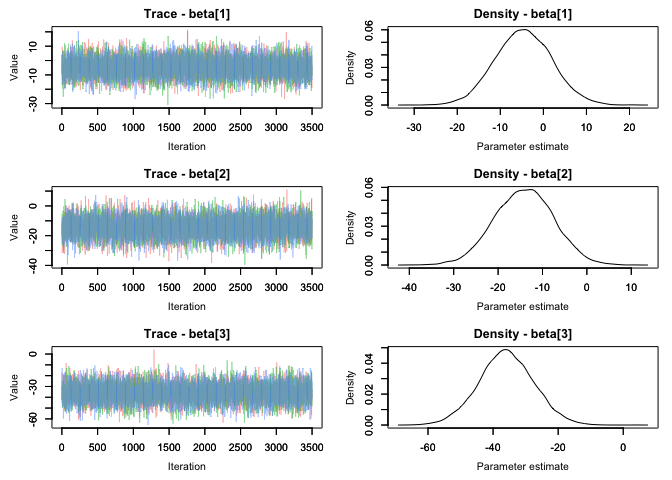Just trace plots can be plotted with `type = 'trace'`. Just density plots can be plotted with `type = 'density'`. Default is `type = 'both'` which outputs both trace and density plots. Density plots for individual chains can be output using the `ind` argument.

``````MCMCtrace(MCMC_data,
params = 'beta',
type = 'density',
ind = TRUE,
pdf = FALSE)``````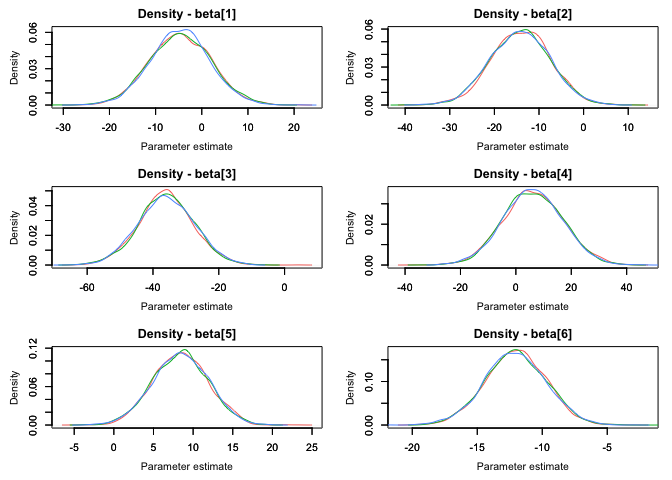The PDF document will be output to the current working directory by default, but another directory can be specified. The `open_pdf` argument can be used to prevent the produced PDF from opening in a viewer once generated.

``````MCMCtrace(MCMC_data,
pdf = TRUE,
open_pdf = FALSE,
filename = 'MYpdf',
wd = 'DIRECTORY_HERE')``````

`iter` denotes how many iterations should be plotted for the chain the trace and density plots. The default is 5000, meaning that the last 5000 iterations of each chain are plotted. Remember, this is the final posterior chain, not including the specified burn-in or warm-up (specified when the model was run). If less than 5000 iterations are run, the full number of iterations will be plotted.

``````MCMCtrace(MCMC_data,
params = c('beta', 'beta', 'beta'),
ISB = FALSE,
exact = TRUE,
iter = 100,
ind = TRUE,
pdf = FALSE)``````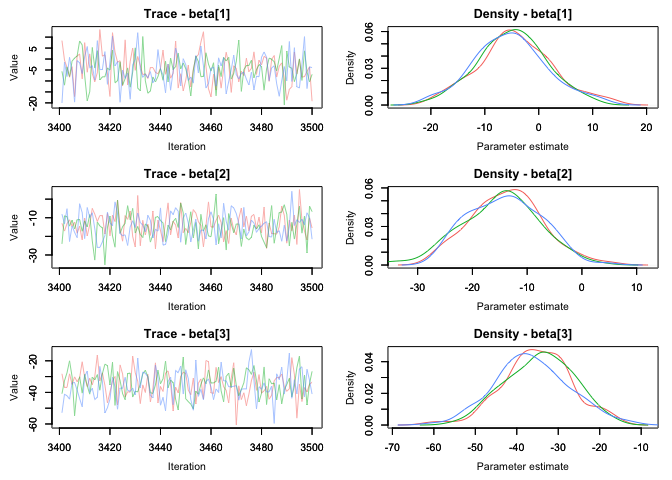Overlap between the priors and posteriors (PPO - prior posterior overlap) can also be calculated by specifying the priors associated with each parameter using the `priors` argument. This is particularly useful when investigating how large the effect of the prior is on the posterior distribution - this can be informative when trying to determine how identifiable a particular parameter is in a model.

The `priors` argument takes a matrix as input, with each column representing a prior for a different parameter and each row representing a random draw from that prior distribution. These draws can be generated using R functions such as rnorm, rgamma, runif, etc. Parameters are plotted alphabetically - priors should be sorted accordingly. If the `priors` argument contains only one prior and more than one parameter is specified for the `params` argument, this prior will be used for all parameters. The number of draws for each prior should equal the number of iterations specified by (or total draws if less than ) times the number of chains, though the function will automatically adjust if more or fewer iterations are specified. It is important to note that some discrepancies between MCMC samplers and R may exist regarding the parameterization of distributions - one example of this is the use of precision in JAGS but standard deviation in R and Stan for the ‘second parameter’ of the normal distribution. Values for Rhat and number of effective samples can be plotting on the density plots using the `Rhat` and `n.eff` arguments.

``````# note that the same prior used for all parameters
# the following prior is equivalent to dnorm(0, 0.001) in JAGS
PR <- rnorm(15000, 0, 32)

MCMCtrace(MCMC_data,
params = c('beta', 'beta', 'beta'),
ISB = FALSE,
exact = TRUE,
priors = PR,
pdf = FALSE,
Rhat = TRUE,
n.eff = TRUE)``````
``````## Warning in MCMCtrace(MCMC_data, params = c("beta", "beta", "beta"), :
## Only one prior specified for > 1 parameter. Using a single prior for all
## parameters.``````
``````## Warning in MCMCtrace(MCMC_data, params = c("beta", "beta", "beta"), :
## Number of samples in prior is greater than number of total or specified
## iterations (for all chains) for specified parameter. Only last 10500 iterations
## will be used.``````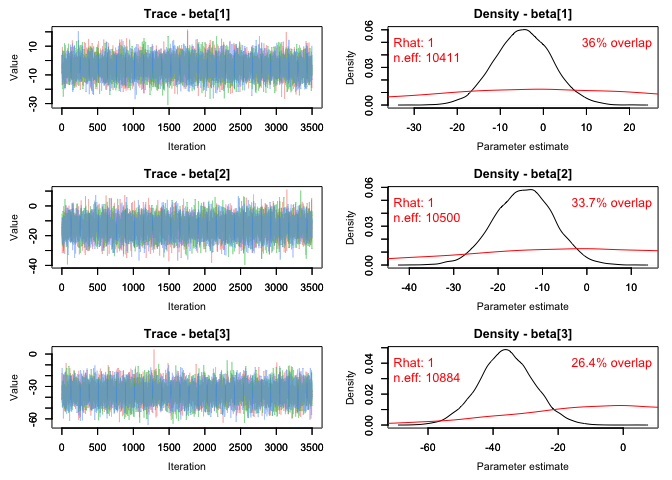Plots can be scaled to visualize both the posterior and the prior distribution using the `post_zm` argument.

``````MCMCtrace(MCMC_data,
params = c('beta', 'beta', 'beta'),
ISB = FALSE,
exact = TRUE,
priors = PR,
pdf = FALSE,
post_zm = FALSE)``````
``````## Warning in MCMCtrace(MCMC_data, params = c("beta", "beta", "beta"), :
## Only one prior specified for > 1 parameter. Using a single prior for all
## parameters.``````
``````## Warning in MCMCtrace(MCMC_data, params = c("beta", "beta", "beta"), :
## Number of samples in prior is greater than number of total or specified
## iterations (for all chains) for specified parameter. Only last 10500 iterations
## will be used.``````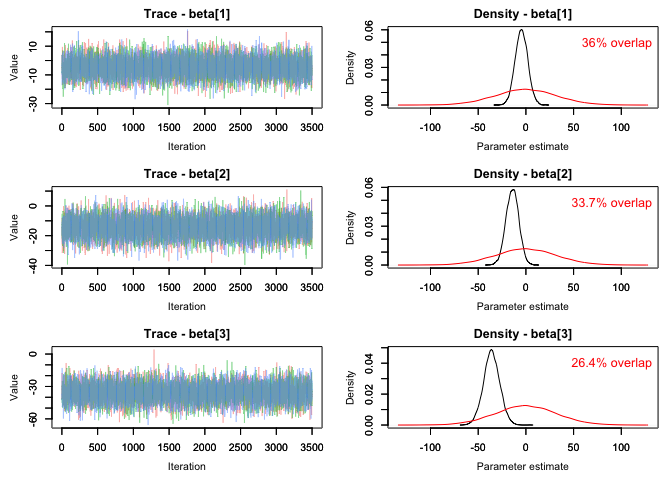Percent overlap can be output to an R object as well using the `PPO_out` argument. Plotting of the trace plots can be suppressed with `plot = FALSE`.

``````PPO <- MCMCtrace(MCMC_data,
params = c('beta', 'beta', 'beta'),
ISB = FALSE,
exact = TRUE,
priors = PR,
plot = FALSE,
PPO_out = TRUE)``````
``````## Warning in MCMCtrace(MCMC_data, params = c("beta", "beta", "beta"), :
## Only one prior specified for > 1 parameter. Using a single prior for all
## parameters.``````
``````## Warning in MCMCtrace(MCMC_data, params = c("beta", "beta", "beta"), :
## Number of samples in prior is greater than number of total or specified
## iterations (for all chains) for specified parameter. Only last 10500 iterations
## will be used.``````
``PPO``
``````##     param percent_PPO
## 1 beta        36.0
## 2 beta        33.7
## 3 beta        26.4``````

Additional arguments can be used to change the limits of the density plots, axes labels, plot titles, line width and type, size and color of text, tick and axes label size, position of ticks, color of lines, and thickness of axes.

``````MCMCtrace(MCMC_data,
params = c('beta', 'beta', 'beta'),
ISB = FALSE,
exact = TRUE,
priors = PR,
pdf = FALSE,
Rhat = TRUE,
n.eff = TRUE,
xlab_tr = 'This is the x for trace',
ylab_tr = 'This is the y for trace',
main_den = 'Custom density title',
lwd_den = 3,
lty_pr = 2,
col_pr = 'green',
sz_txt = 1.3,
sz_ax = 2,
sz_ax_txt = 1.2,
sz_tick_txt = 1.2,
sz_main_txt = 1.3)``````
``````## Warning in MCMCtrace(MCMC_data, params = c("beta", "beta", "beta"), :
## Only one prior specified for > 1 parameter. Using a single prior for all
## parameters.``````
``````## Warning in MCMCtrace(MCMC_data, params = c("beta", "beta", "beta"), :
## Number of samples in prior is greater than number of total or specified
## iterations (for all chains) for specified parameter. Only last 10500 iterations
## will be used.``````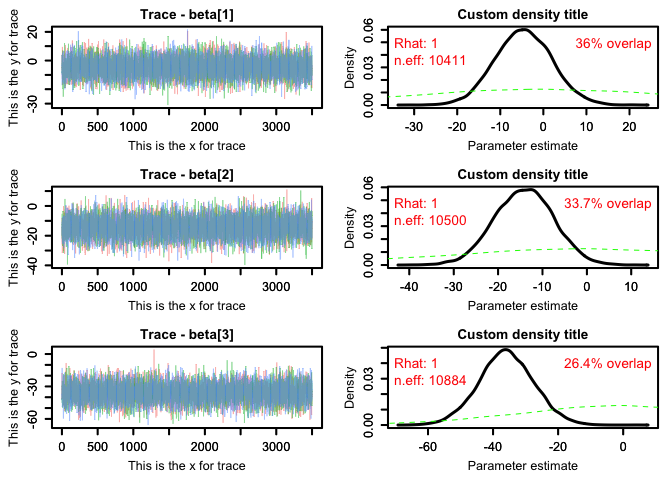If simulated data were used to fit the model, the generating values used to simulate the data can be specified using the `gvals` argument. This makes it possible to compare posterior estimates with the true parameter values. Generating values will be displayed as vertical dotted lines. Similar to the `priors` argument, if one value is specified when more than one parameter is used, this one generating value will be used for all parameters.

``````# generating values for each parameter used to simulate data
GV <- c(-10, -5.5, -15)

MCMCtrace(MCMC_data,
params = c('beta', 'beta', 'beta'),
ISB = FALSE,
exact = TRUE,
gvals = GV,
pdf = FALSE)``````